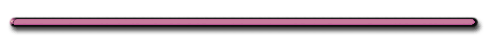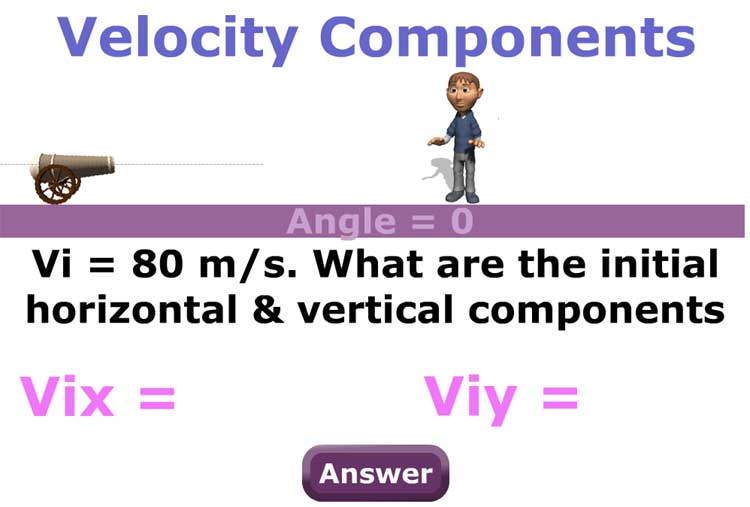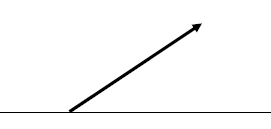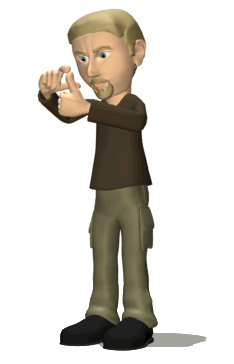How many things would you have
to get right to make this work?

2. Projectile fired at an angle (not 0 or 90)

 Projectile Path is Parabolic Baseball Throw showmethephysics.com Victoria Lollo - Class of 2007

Angle and Intitial Velocities

- Projectile fired at 5 m/s:

if Ө = 0

Vix = 5 m/s,  Viy = 0

if Ө = 90

then .....

Vix= 0 m/s and Viy = 5 m/s

If angle not 0 or 90 the  initial velocity is part vertical and part horizontal

[ Flash ]
St. Mary's H.S. Physics[ Flash ]
St. Mary's H.S. Physics

*** To understand this type of motion we must breakup the velocity into horizontal and vertical parts (components)

Finding Initial Horizontal & Vertical Components (parts)

 [ Flash ]

V - velocity of projectile fired

Vix - initial V in x direction

Viy - initial V in y direction

Use Trig. to Find Vix and Viy

Since…

COSӨ = A/H = Vix/V

 [ Flash ]

CROSS MULTIPLY:

Initial Horizontal
Component of Velocity

 Vix = VcosӨ (on reference table)

Since…

sinӨ = Opp./Hyp. = Viy/V

CROSS MULTIPLY:

Initial Vertical
Component of Velocity

 Viy = VsinӨ (on reference table)During the times the ball is in the air,
how many times is the vertical velocity zero?A bouncing ball captured with a stroboscopic flash at 25 images per second.

MichaelMaggs Edit by Richard Bartz

2 times / At the Peak

 Ex) A baseball is thrown upward at an angle of 30.° and a velocity of 6.0 m/s. Find the initial horizontal & vertical components of the baseball's velocity.Vertical Component

Viy = VsinӨ

=(6.0 m/s)sin30.°

Viy = 3.0 m/s up

Horizontal Component

Vix = VcosӨ

= (6.0 m/s)cos30.°

Vix = 5.2 m/s across

3. Other Important Facts

dx = Vxt

(memorize)

 ay = -9.8 m/s2 Ascending (down -) ay =+9.8 m/s2 Descending (down +) Vy at Peak = 0

Angles and Ranges

What angle produces the greatest range?

45 degreesDon IonName another angle
that will give the same range (dx)

55 degrees

35 degrees

65 degrees

25 degrees

55 degrees

35 degrees

20 degrees

70 degrees

Minimum Range

0°, 90°Maximum Range

45°Angle with Longest
Time in Air?

90 degrees[ HTML5 ]
St. Mary's H.S. PhysicsEx) Which angle of projection causes a projectile to stay in the air longer?    50 or 70 degrees?

70 degrees,

(closest to 90,
longer time in air)

 RankingTask 1.   A rock is thrown at various angles but with the same initial velocity. 2°    70°    20°   5°   60°   Rank the angles above from smallest horizontal displacement (dx) to the greatestProjectile at an Angle- Word Problems

[AP]